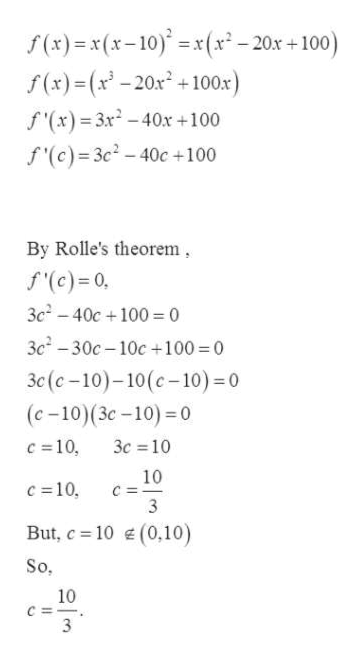# Describe whether Rolle's theorem applies to the function shown below on the given interval. If so, find the point(s) that are guaranteed to exist by Rolle's theorem.f(x)=x(x-10)^2 ; [0,10]

Question

Describe whether Rolle's theorem applies to the function shown below on the given interval. If so, find the point(s) that are guaranteed to exist by Rolle's theorem.

f(x)=x(x-10)^2 ; [0,10]

check_circle

Step 1

Given:

Describe whether Rolle's theorem applies to the function shown below on the given interval. If so, find the point(s) that are guaranteed to exist by Rolle's theorem.

Step 2

Rolle’s Theorem:  Let f be continuous on the closed interval [a, b] and differentiable on the open interval (a, b). If f (a) = f (b), then there is at least one number c in (a, b) such that f’(c) = 0.

Step 3

Now since:

1. f(x) is a polynomial function so it is continuous everywhere in the given interval.
2. f’(x) is also differentiable in given open interval.
3. f (0) = f (10) = 0.

Hence all the hypotheses are true for the given fu...help_outlineImage Transcriptionclosef(x)x(x-10)x(x-20x + 100) f(x)=(x-20x+100x) f '(x)= 3x2-40x +100 f'(c)=3c2- 40c+100 By Rolle's theorem f'(c)= 0 3c-40c100 0 3c-30c-10c+100 0 3c(c-10)-10(c-10) 0 (c-10)(3c-10) 0 c 10 3c 10 10 c 10 3 (0,10) But, c 10 So 10 C 3 fullscreen

### Want to see the full answer?

See Solution

#### Want to see this answer and more?

Solutions are written by subject experts who are available 24/7. Questions are typically answered within 1 hour.*

See Solution
*Response times may vary by subject and question.
Tagged in

### Calculus